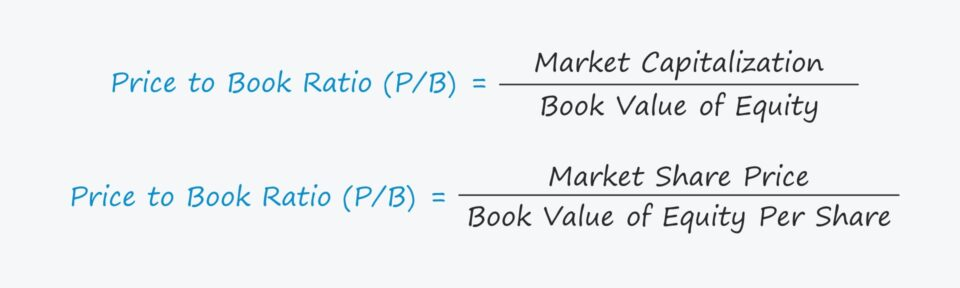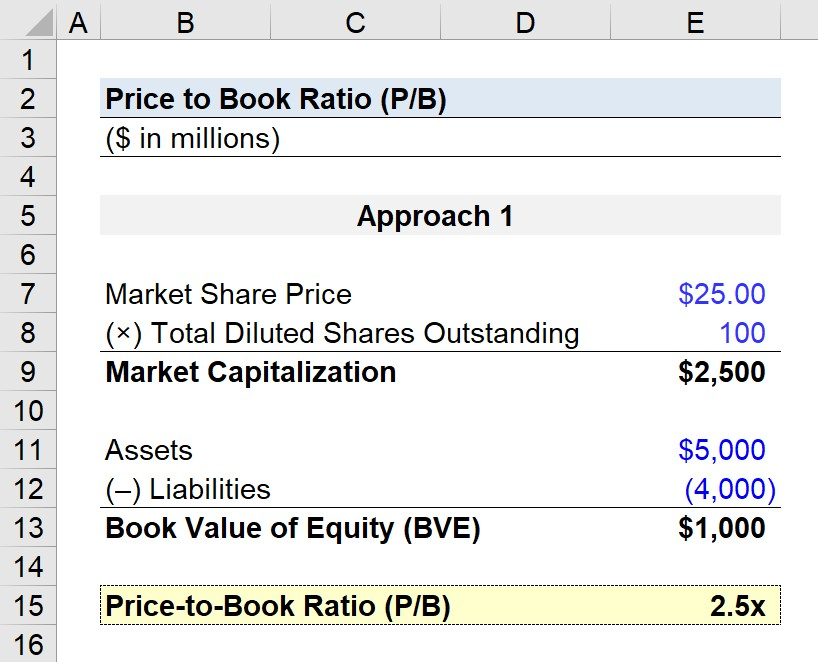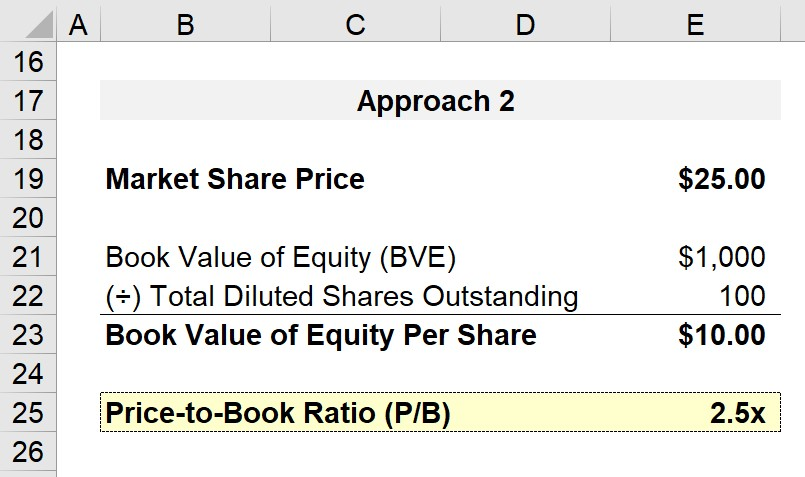Welcome to Wall Street Prep! Use code at checkout for 15% off.# Price to Book (P/B Ratio)

Guide to Understanding the Price to Book Ratio (P/B)## How to Calculate Price to Book Ratio (P/B)?

The price to book ratio, often abbreviated as the “P/B ratio”, compares the current market capitalization (i.e. equity value) to its accounting book value.

• Market Capitalization → The market capitalization is calculated as the current share price multiplied by the total number of diluted shares outstanding. Conceptually, the market cap represents the pricing of a company’s equity according to the market, i.e. what investors currently believe the company to be worth.
• Book Value (BV) → The book value (BV) on the other hand, is the net difference between the carrying asset value on the balance sheet less the company’s total liabilities. The book value reflects the value of the assets that a company’s shareholders would receive if the company was hypothetically liquidated (and the book value of equity is an accounting metric, rather than based on the market value).

Since the book value of equity is a levered metric (post-debt), the equity value is used as the point of comparison, rather than the enterprise value, to avoid a mismatch in the represented capital provider(s).

For the most part, any financially sound company should expect its market value to be greater than its book value, since equities are priced in the open market based on the forward-looking anticipated growth of the company.

If the market valuation of a company is less than its book value of equity, that means the market does not believe the company is worth the value on its accounting books. Yet in reality, a company’s book value of equity is seldom lower than its market value of equity, barring unusual circumstances.

## Price to Book Ratio Formula (P/B)

The price to book ratio (P/B) is calculated by dividing a company’s market capitalization by its book value of equity as of the latest reporting period.

Price to Book Ratio (P/B) = Market Capitalization ÷ Book Value of Equity

Or, alternatively, the P/B ratio can also be calculated by dividing the latest closing share price of the company by its most recent book value per share.

Price to Book Ratio (P/B) = Market Share Price ÷ Book Value of Equity Per Share

## What is a Good Price to Book Ratio?

The norm for the P/B varies by industry, but a P/B ratio under 1.0x tends to be viewed favorably and as a potential indication that the company’s shares are currently undervalued.

While P/B ratios on the lower end can generally suggest a company is undervalued and P/B ratios on the higher end can mean the company is overvalued — a closer examination is still required before any investment decision can be made. From a different perspective, underperformance can lead to lower P/B ratios, as the market value (i.e. the numerator) should rightfully decrease.

• P/B Ratio < 1.0x → A sub-1.0x P/B ratio should NOT be immediately interpreted as a sign that the company is undervalued (and is an opportunistic investment). In fact, a low P/B ratio can indicate problems with the company that could lead to value deterioration in the coming years (i.e. a “red flag”).
• P/B Ratio > 1.0x → Companies with P/B ratios far exceeding 1.0x could be a function of recent positive performance and a more optimistic outlook on the company’s future outlook by investors.

The price to book ratio is more appropriate for mature companies, like the P/E ratio, and is especially accurate for those that are asset-heavy (e.g. manufacturing, industrials).

The P/B ratio is also typically avoided for companies composed mostly of intangible assets (e.g. software companies) since most of their value is tied to its intangible assets, which are not recorded on a company’s books until the occurrence of an event such as an acquisition.

## Price to Book Ratio Summary: Definition, Description and IssuesPrice to Book Value (P/B) Ratio Commentary Slide (Source: WSP Trading Comps Course)

## Price to Book Ratio Calculator (P/B)

We’ll now move to a modeling exercise, which you can access by filling out the form below.Submitting...

## 1. Price to Book Ratio Calculation Example (Market Cap Approach)

For our example exercise calculating the P/B ratio, we’ll be going through the steps for the two approaches we mentioned earlier.

The shared assumptions are listed below:

• Latest Closing Share Price = \$25.00
• Total Diluted Shares Outstanding = 100 million

With those two provided metrics, we can calculate the market capitalization as \$2.5bn

• Market Capitalization = Latest Closing Share Price × Total Diluted Shares Outstanding
• Market Capitalization = \$25.00 × 100 million = \$2.5 billion

Now that the calculation for the numerator is done, we can now move to the denominator.

The assumptions for the book value of equity can be found below:

• Assets = \$5 billion
• Liabilities = \$4 billion

Upon subtracting Liabilities from Assets, we can calculate the book value of equity (BVE).

• Book Value of Equity (BVE) = Assets – Liabilities
• BVE = \$5 billion – \$4 billion = \$1 billion

The final step of our price to book ratio calculation under the first approach is to divide our company’s market cap by its book value of equity (BVE).

• P/B Ratio = Market Capitalization ÷ Book Value of Equity
• P/B Ratio = \$2.5 billion ÷ \$1 billion = 2.5x## 2. P/B Ratio Calculation Example (Share Price Approach)

In the next part of our exercise, we’ll calculate the P/B ratio using the share price approach, so the corresponding metric is the book value of equity per share (BVPS).

Since we already have the latest closing share price, the only remaining step is to adjust the book value of equity (BVE) to a per-share basis.

• Book Value Per Share (BVPS) = \$1 billion ÷ \$100 million = \$10.00

In our last step, we’ll divide the current share price by the BVE per share.

• P/B Ratio = Latest Closing Share Price ÷ Book Value Per Share
• P/B Ratio = \$25.00 ÷ \$10.00 = 2.5xLike the first approach, in which we divided the market capitalization by the book value of equity, we arrive at a P/B ratio of 2.5x.

In conclusion, whether the company is undervalued, fairly valued, or overvalued will depend on how the company’s ratios compare with the industry average multiples, as well as the fundamentals of the company.

To reiterate from earlier, the P/B ratio is a screening tool for finding potentially undervalued stocks, but the metric should always be supplemented with in-depth analyses of the underlying value drivers.Step-by-Step Online Course

### Everything You Need To Master Financial Modeling

Enroll in The Premium Package: Learn Financial Statement Modeling, DCF, M&A, LBO and Comps. The same training program used at top investment banks.Inline FeedbacksLearn Financial Modeling Online

Everything you need to master financial and valuation modeling: 3-Statement Modeling, DCF, Comps, M&A and LBO.

X

The Wall Street Prep Quicklesson Series

7 Free Financial Modeling Lessons

Get instant access to video lessons taught by experienced investment bankers. Learn financial statement modeling, DCF, M&A, LBO, Comps and Excel shortcuts.